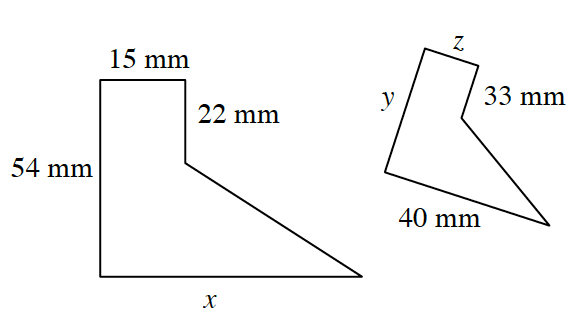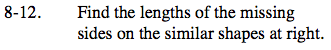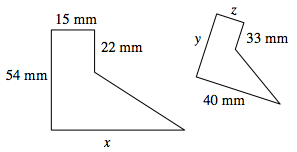Home > ACC7 > Chapter cc38 > Lesson cc38.1.1 > Problem8-12

8-12.. Find the lengths of the missing sides on the similar shapes at right. Homework Help ✎Similar shapes have sides with equal ratios to each other. To find each unknown side, compare its ratio to a known side.For example, the ratio of the 15 mm side to the 22 mm side should be equal to the ratio of the z side to the
33 mm side.

$\frac{15}{22}=\frac{\it z}{33}$

z = 22.5 mm

You can now use the value of z to find ratios for the other unknown sides. Write two more ratios to solve for side x and side y.

$\frac{15}{54}=\frac{22.5}{\it y}$

15y = (22.5) (54)

y = 81 mm
What does x equal?